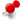How to create a figure with two graphviz diagrams in it?ClassicListThreaded3 messagesOpen this post in threaded view
|

How to create a figure with two graphviz diagrams in it?

 Hello, I want to create a figure containing two graphviz diagrams. How can I do this in asciidoc? [graphviz] .... digraph A { } .... [graphviz] .... digraph B { } .... I want the diagrams above to be part of a single figure. Sid